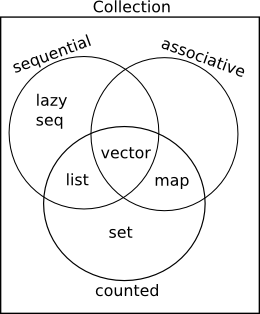## 数据结构

### Collection 和 Sequence

Clojure 常用的数据结构有 List, Map, Vector, Set. 他们都属于 Collection, 之间的关系大致是这样:Clojure 中的数据结构被设计成不可变的, 用来配合函数式编程. 这和主流编程语言存在区别.

### Sequence & List

Clojure 中的 List 是单链表. 适合从头部访问, 而不适合随机访问. Clojure 采用 Lisp 语法来定义 List:

``````'(1 2 3 4)
; (1 2 3 4)
(list? '(1 2 3 4))
; true
``````

``````(quote (1 2 3 4))
; (1 2 3 4)
``````

``````(list 1 2 3 4)
; (1 2 3 4)
``````

Sequence 是一种抽象的数据结构, List 本身是一种 Sequence, 同时 Sequence 本身可以表示惰性的列表.

``````(type (range))
; cljs.core/Range
(seq? (range))
; true
``````

``````(seq [1 2 3])
; (1 2 3)
``````

``````(first (list 1 2 3 4))
; 1
(rest (list 1 2 3 4))
; (2 3 4)
(cons 1 '(2 3 4 5 6))
; (1 2 3 4 5 6)
``````

### Vector

Vector 支持随机访问, 通过 `[]` 语法可以快速定义:

``````[1 2 3 4]
; [1 2 3 4]
(vector? [])
; true
``````

``````(vector 1 2 3 4)
; [1 2 3 4]
(vec '(1 2 3 4))
; [1 2 3 4]
``````

``````(get [:a :b :c :d] 1)
; :b
(nth [:a :b :c :d] 1)
; :b
(peek [1 2 3 4])
; 4
(pop [1 2 3])
; [1 2]
(conj [1 2 3] 4)
; [1 2 3 4]
(subvec [1 2 3] 1 2) ; 截取向量
; 
``````

### Map

Map 是关联列表, 可以通过 `{}` 语法定义 Map, 其中 `,` 等同于空白. 一般键和值的类型没有具体限制:

``````{:a "a", "b" 3, 4 []}
; {:a "a", "b" 3, 4 []}
(map? {})
; true
``````

``````{:name "Clojure", :born 2007}
``````

``````(hash-map 1 2 3 4)
; {1 2, 3 4}
(zipmap [:a :b :c] [1 2 3])
; {:a 1, :b 2, :c 3}
``````

``````(:a {:a 1})
; 1
(assoc {:a 1, :b 2} :c 3)
; {:a 1, :b 2, :c 3}
(dissoc {:a 1} :b 2)
; {:a 1}
(contains? {:a 1} :b)
; false
(merge {:a 1} {:b 2})
; {:a 1, :b 2}
(update {:a 1} :a inc)
; {:a 2}
``````

### Set

``````#{1 2 3}
; #{1 2 3}
(set? #{})
; true
``````

``````(hash-set 1 2 3)
; #{1 2 3}
(set [1 2 3])
; #{1 2 3}
``````

``````(conj #{1 2 3} 4)
; #{1 2 3 4}
(disj #{1 2 3} 3)
; #{1 2}
(contains? #{1 2 3} 4)
; false
``````

### 常用函数

• `into`

``````(into [] '(1 2 3))
; [1 2 3]
(into #{} '(1 2 3))
; #{1 2 3}
(into '() [1 2 3])
; (3 2 1)
(into {} [[:a 1] [:b 2]])
; {:a 1, :b 2}
``````
• `first`

`first` 取出 Sequence 的第一个元素, 但是对于 Map 来说能获取键值对:

``````(first [1 2 3])
; 1
(first '(1 2 3))
; 1
(first #{1 2 3})
; 1
(first {:a 1 :b 2})
; [:a 1]
``````
• `map`

``````(map inc [1 2 3])
; (2 3 4)
(map inc '(1 2 3))
; (2 3 4)
(map inc #{1 2 3})
; (2 3 4)
(map identity {:a 1 :b 2})
; ([:a 1] [:b 2])
``````

``````(mapv identity {:a 1 :b 2})
; [[:a 1] [:b 2]]
(into [] (map identity {:a 1 :b 2}))
; [[:a 1] [:b 2]]
``````
• `peek`, `pop``conj`

``````(peek [1 2 3])
; 3
(peek '(1 2 3))
; 1
(pop '(1 2 3))
; (2 3)
(pop [1 2 3])
; [1 2]
(conj [1 2 3] 4)
; [1 2 3 4]
(conj '(1 2 3) 4)
; (4 1 2 3)
``````
• `count`
``````(count '(1 2 3))
; 3
(count [1 2 3])
; 3
(count "123")
; 3
(count #{1 2 3})
; 3
(count {:a 1 :b 2 :c 3})
; 3
``````

``````(empty? [])
; true
(empty? {})
; true
(empty? #{})
; true
(empty? '())
; true
``````
• `assoc-in``update-in`

``````(get-in {:a [1 2 3]} [:a 0])
; 1
(assoc-in {:a [1 2 3]} [:a 0] 4)
; {:a [4 2 3]}
(update-in {:a [1 2 3]} [:a 0] inc)
; {:a [2 2 3]}
``````